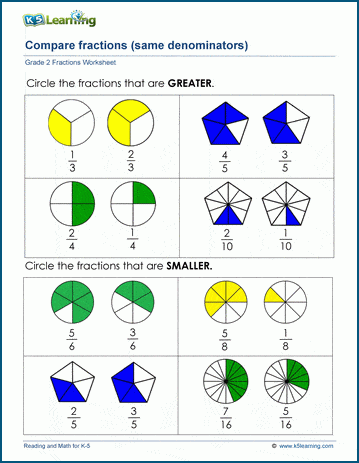# Comparing fractions (same denominator)

## Math worksheets: Comparing fractions with the same denominator

These grade 2 math worksheets use pictures to help students decide which fraction is larger or smaller.  All fractions have the same denominator, meaning that all pictured shapes are divided into the same number of equal parts.  Students will learn that if the denominator is the same, the fraction with the larger numerator is greater.## More fractions worksheets

Explore all of our fractions worksheets, from dividing shapes into "equal parts" to multiplying and dividing improper fractions and mixed numbers.

## What is K5?

K5 Learning offers reading and math worksheets, workbooks and an online reading and math program for kids in kindergarten to grade 5.  We help your children build good study habits and excel in school.